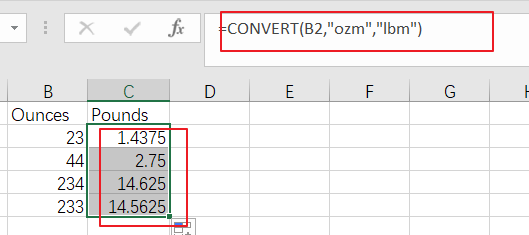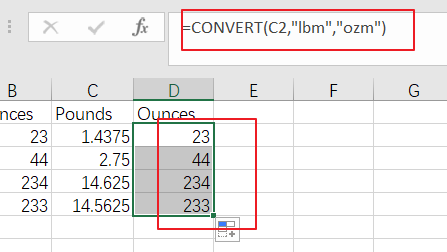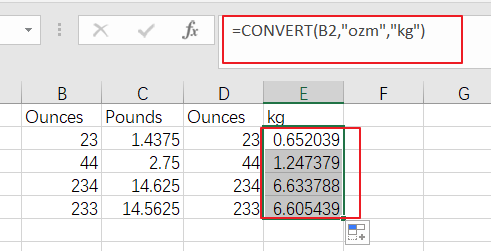# How to Convert Ounces to Pounds or Grams in Excel

This post will guide you how to convert ounces to pounds or grams with formula in Excel. How do I convert pounds to ounces or grams in Excel 2013/2016.

## Convert Ounces to Pounds

Assuming that you have a list of data in range B1:B4, in which contain values with Ounces data, and you wish to convert these Ounces to pounds. How to do it. You can use an formula based on the CONVERT function to convert it quickly. Like this:

`=CONVERT(B2,"ozm","lbm")`

You need to type this formula into a blank cell such as: Cell C2, and press Enter key on your keyboard, and then drag the AutoFill handle down to other cells to apply this formula.## Convert Pounds to Ounces

If you want to convert your range of cells with Pounds data to Ounces, you can use the below formula based on the CONVERT function:

`=CONVERT(C2,"lbm","ozm")`

You need to type this formula into a blank cell such as: Cell D2, and press Enter key on your keyboard, and then drag the AutoFill handle down to other cells to apply this formula.## Convert Ounces to kg

If you want to convert kg into Ounces for you range B2:B5, just use the following formula:

`=CONVERT(B2,"ozm","kg")`

You need to type this formula into a blank cell such as: Cell E2, and press Enter key on your keyboard, and then drag the AutoFill handle down to other cells to apply this formula.Related Posts
If Cell Contains Either Text1 or Text2

IF function is frequently used in Excel worksheet to return “true value” or “false value” based on the logical test result. If you want to see if cell contains certain substring1 like “abc” or substring2 like “def”, and returns true ...

If Cell Contains Certain Text OR Equals Certain Text

IF cell equals certain text IF function is frequently used in Excel worksheet to return “true value” or “false value” based on the logical test result. If you want to test values to see if they equal certain text like ...

VLOOKUP From Another Sheet Not Working

In the previous post, you should know that how to fix or remove the #N/A error when using VLOOKUP formula to lookup value from another sheet. And this post will show you reasons why your VLOOKUP formula is not working ...

If Cell Begins with One of Three Supplied Characters

If you want to test values to see if they begin with some given specific characters like “x”, ”y”, or “z”, you can create a formula with COUNTIF and SUM functions to return results. EXAMPLE You can see “TRUE” or ...

Fix #N/A Error For VLOOKUP From Another Sheet

This post will show you how to fix the #N/A error why it occurs when you extract values from another sheet using VLOOKUP function in Excel 2016,2013,2010 or other Excel versions. How can you correct a #N/A error in VLOOKUP ...

How To Remove Special Characters in Excel

This post will show you how to remove special characters from text strings in Excel. And I am going to introduce two ways to remove special characters (@&)#%\$) from a string in Excel. Removing Special Characters using Power Query If ...

How To Insert Comments in Protected Worksheet in Excel

This post will show you how to allow comments in a protected worksheet in Excel. You can easily to insert comments into cells in a normal worksheet in Excel, but if want to insert a comment in a worksheet that ...

How To Convert Text to Upper Cases(Using VBA) in Excel

This post will show you how to switch from lower case to upper case in Excel. and I am going to show you two different ways of converting text to upper cases using formula or VBA macro in Excel 2013,Excel ...

How To Hide Every Other Row in Excel (Using VBA)

This post will show you how to hide alternate rows or columns in Excel or how to hide every third, fourth, fifth row or column in Excel. If you want to hide every other row in your current worksheet, how ...

How to Average Only Positive or Negative Numbers of a Range

Suppose both positive numbers and negative numbers exist in a table. If we want to know the average of only positive numbers in this table, we can create a formula to get average of all positive numbers with all negative ...

Sidebar